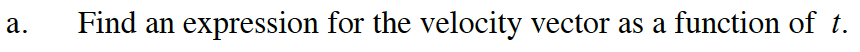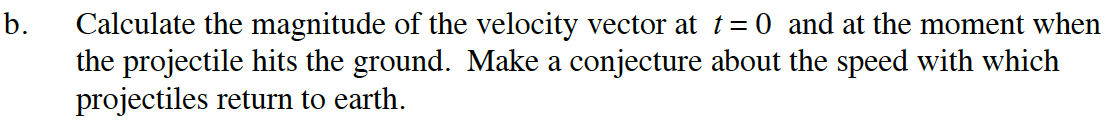### Home > CALC > Chapter 12 > Lesson 12.2.1 > Problem12-67

12-67.
1. A projectile is launched from the ground at a 45° angle with altitude (in feet after t seconds) given by y(t) = 96t − 16t2 . Its horizontal displacement in feet is given by x(t) =96t. Homework Help ✎

1. Find an expression for the velocity vector as a function of t.

2. Calculate the magnitude of the velocity vector at t = 0 and at the moment when the projectile hits the ground. Make a conjecture about the speed with which projectiles return to earth.To determine when the projectile hits the ground, solve 0 = 96t – 16t2.

The velocity vector when t = 0 is:

Sketch this vector and use the Pythagorean Theorem to determine its magnitude.